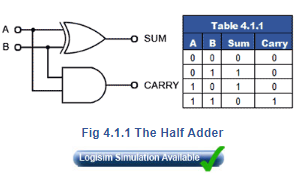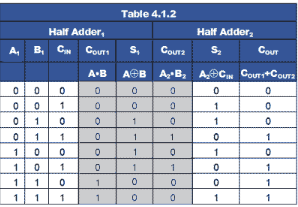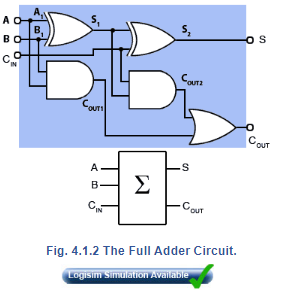# Half Adder And Full Adder Electrical Engineering (EE) Notes | EduRev

## Electrical Engineering (EE) : Half Adder And Full Adder Electrical Engineering (EE) Notes | EduRev

The document Half Adder And Full Adder Electrical Engineering (EE) Notes | EduRev is a part of the Electrical Engineering (EE) Course Digital Electronics.
All you need of Electrical Engineering (EE) at this link: Electrical Engineering (EE)

Binary Arithmetic  is carried out by combinational logic circuits, the simplest of which is the half adder, shown in Fig. 4.1.1. This circuit consists, in its most basic form of two gates, an XOR gate  that produces a logic 1 output whenever A is 1 and B is 0, or when B is 1 and A is 0. The AND gate produces a logic 1 at the carry output when both A and B are 1. The half adder truth table is shown in Table 4.1.1 and describes the result of binary addition.1 plus 0 = 12 (110)

and

1 plus 1 = 102 (210)

The half adder is fine for adding two 1-bit numbers together, but for binary numbers containing several bits, a carry may be produced at some time (as a result of adding 1 and 1) that must be added to the next column. As the half adder has only two inputs it cannot add in a carry bit from a previous column, so it is not practical for anything other than 1-bit additions.When 2 or more bits are to be added, the circuit used is the Full Adder, shown in Fig 4.1.2, (blue background) together with its simplified block diagram symbol. This circuit simply comprises two half adders, the sum of A and B from the first half adder is used as input A on the second half adder, which now produces a sum of the first half adder sum (S1) plus any ‘carry in’ from the CIN terminal. Any carries produced by the two half adders are then ‘ORed’ together to produce a single COUT. output. The truth table for the circuit is given in Table 4.1.2.Offer running on EduRev: Apply code STAYHOME200 to get INR 200 off on our premium plan EduRev Infinity!

## Digital Electronics

91 videos|75 docs|8 tests

,

,

,

,

,

,

,

,

,

,

,

,

,

,

,

,

,

,

,

,

,

;